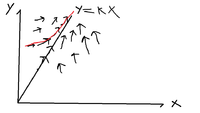# On finding an equation that is made by joining the vectors in a differential vector field

#### Seyed2001

##### New member
Consider the equation dy/dx = cx/y, where c is a real number. Now, consider the points on a Cartesian graph and some vectors associated with each point in such a way that the gradient of each vector is equal to dy/dx. Then, all the vectors of the points below the y=kx (where k is the square root of c) have a gradient more than k and all the vectors of the points above the line have a gradient less than k. The points on the line have their vectors with gradient being equal to k. Now, I start with a point above the line, and let the y and x values change in the direction of the vectors in this field. This generates a curve that its asymptote is the y=kx line. My question is: how can I find an equation for dy/dx in terms of t, where t is a unit of length in the curve (i.e. t is the Pythagorean sum of x and y)?I didn't know how to write equations and powers and etc. so sorry for any inconvenience in understanding my question.

#### HallsofIvy

##### Elite Member
If $$\displaystyle \frac{dy}{dx}= \frac{cx}{y}$$ then $$\displaystyle ydy= cxdx$$ so, integrating, $$\displaystyle \frac{cx^2}{2}= \frac{y^2}{2}+ C$$ which is the same as $$\displaystyle \frac{cx^2}{2}- \frac{y^2}{2}= C$$, a family of hyperbolas all having (0, 0) as center and $$\displaystyle cx^2- y^2=(\sqrt{c}x- y)(\sqrt{c}x+ y)= 0$$ as asymptotes.

•topsquark